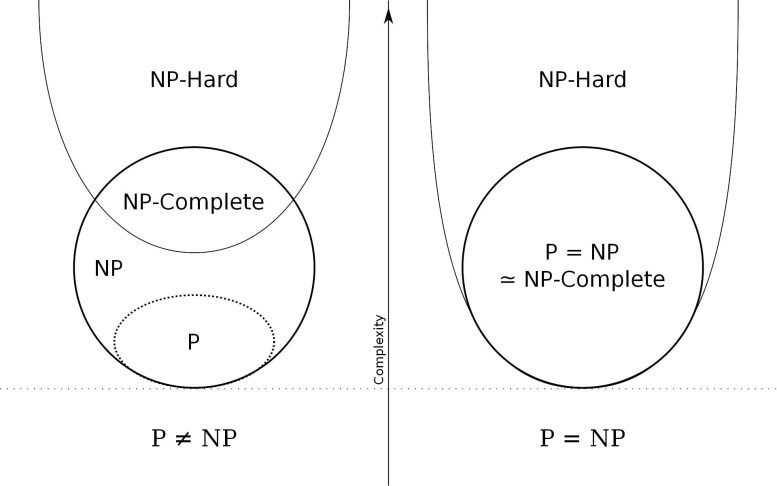# The Million Dollar Problem That Could Break CryptographyThe P vs NP problem is one of the most difficult problems in theoretical computer science.

### P versus NP

Usually, you can verify a solution to a problem. Whether it’s using multiplication for division or plugging the answer in for a variable, math teachers tell you to check your work using your answer in every school math class.

But let’s say you can verify a solution easily, is it just as easy to solve for that solution?

This is the P versus NP problem, a Millenium Prize Problem where the solver will receive a million dollars if valid proof is provided.

#### What is P versus NP?

In computer science, the efficiency of algorithms is very important. Most algorithms are believed to be “fast” if solvable in a standard called polynomial time. Polynomial time is when a problem is solvable in steps scaled by a factor of a polynomial given the complexity of input. So let’s say the complexity of input is some number n, a polynomial time algorithm will be able to solve a problem in nk steps.

Essentially, P vs NP is asking the question: Are problems that can have solutions verified in polynomial time, also have their answers solved in polynomial time?

#### NP-CompletenessAn Euler Diagram showing the cases for NP-Completeness for P ≠ NP and P = NP. Credit: Behnam Esfahbod, Wikimedia Commons (CC-BY 3.0)

One of the most prominent subproblems is NP-complete problems. NP-complete problems are ones that can be verified quickly and that can be used to simulate every other NP-complete problem. Therefore, solving one of these problems in polynomial time is a major boost to solving P vs NP. Some of these problems include games like Battleship and the optimal solution to an NxNxN Rubik’s Cube but also include famous theoretical questions like the traveling salesman problem. If a solution for any of these is found, a general solution for NP-complete problems can also be found.

#### Impact

If P is proven to equal NP, there could be serious consequences and benefits. Cybersecurity would be a huge issue as public-key cryptography would be upended and many ciphers could be cracked. However, there would also be improvements in research of protein structure prediction and overall computing because of better integer programming and the solving of the traveling salesman problem.

If P is proven to not equal NP, there would be nearly no drawbacks and benefits. Researchers would then focus less on a general solution to all NP-complete problems which would not really change much.

#### Closing

P vs NP is a critical unsolved problem in computer science that could have drastic effects. Though the major consensus is that P is not equal to NP, any widely accepted proof would rattle the scientific world.

References:

1. “The P vs NP Problem” by Stephen Cook, 2001, claymath.org
2. “Computers and Intractability: A guide to the Theory of NP-Completeness” by Michael Garey, David S Johnson, 1979, ISBN 0-7167-1045-5